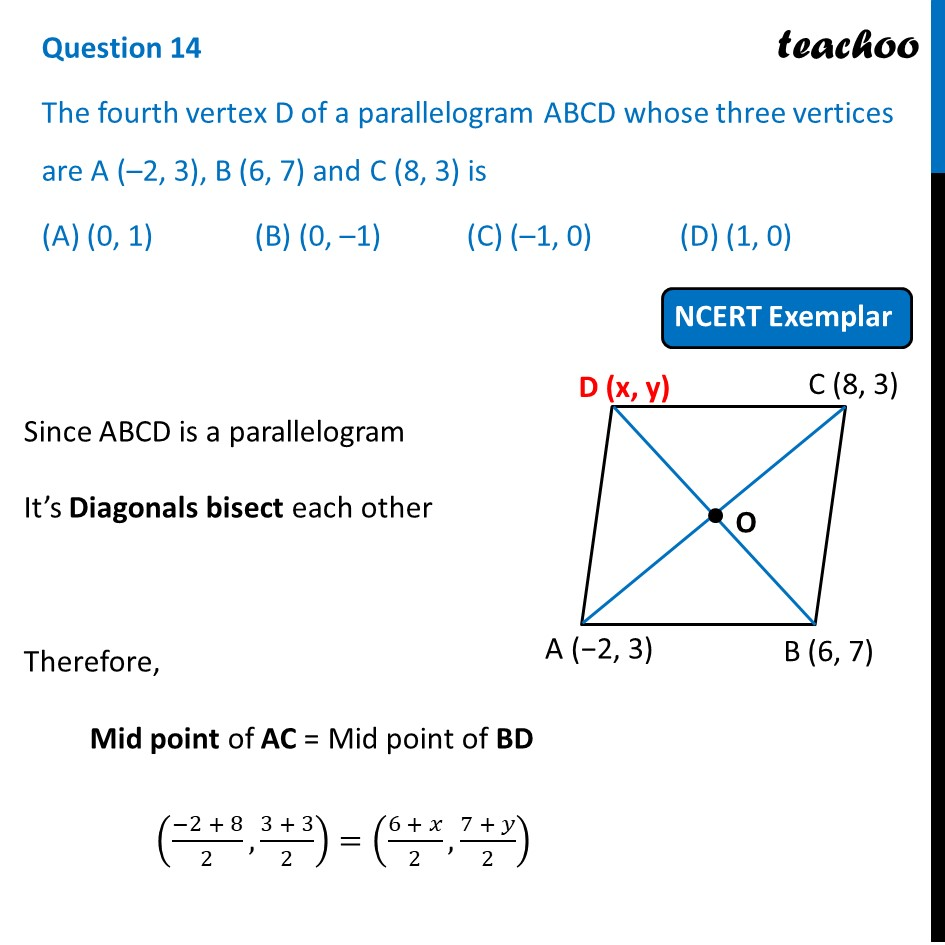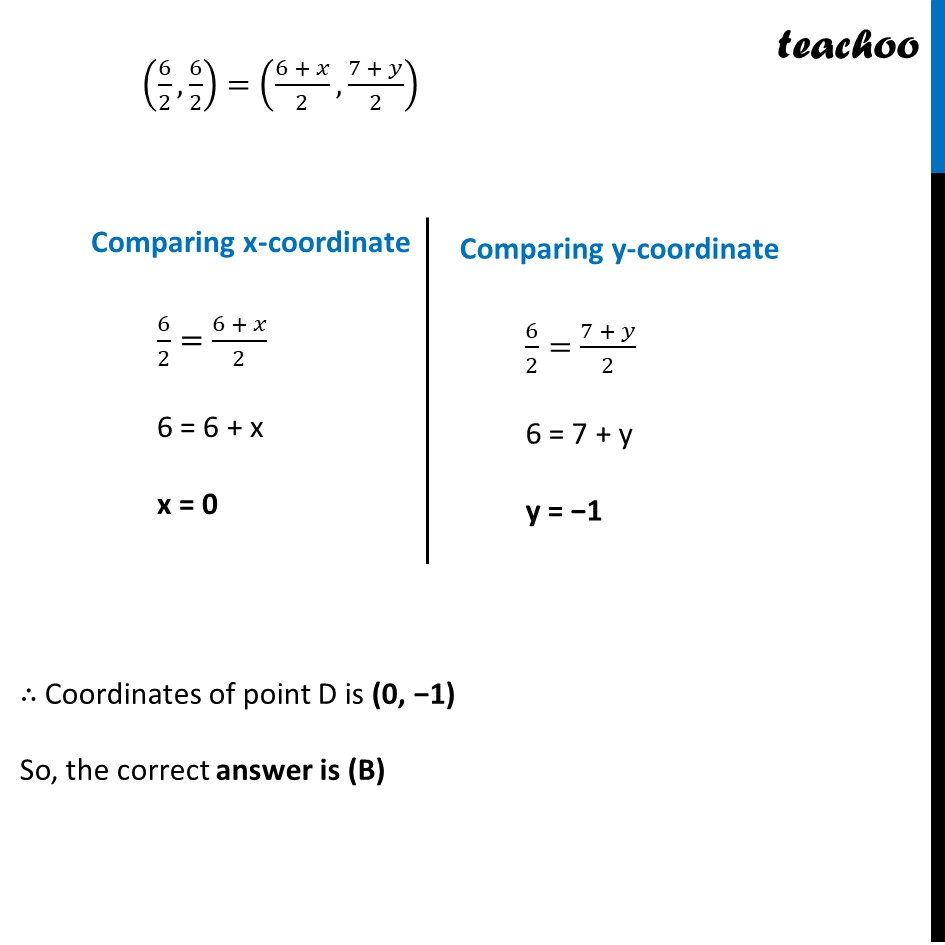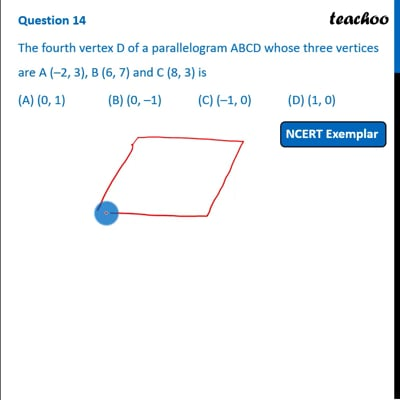NCERT Exemplar - MCQ

Chapter 7 Class 10 Coordinate Geometry
Serial order wise

## (A) (0, 1)   (B) (0, –1)   (C) (–1, 0)   (D) (1, 0)This video is only available for Teachoo black users

Learn in your speed, with individual attention - Teachoo Maths 1-on-1 Class

### Transcript

Question 14 The fourth vertex D of a parallelogram ABCD whose three vertices are A (–2, 3), B (6, 7) and C (8, 3) is (A) (0, 1) (B) (0, –1) (C) (–1, 0) (D) (1, 0) Since ABCD is a parallelogram It’s Diagonals bisect each other Therefore, Mid point of AC = Mid point of BD ((−2 + 8)/2,(3 + 3)/2)=((6 + 𝑥)/2,(7 + 𝑦)/2) (6/2,6/2)=((6 + 𝑥)/2,(7 + 𝑦)/2) Comparing x-coordinate 6/2=(6 + 𝑥)/2 6 = 6 + x x = 0 Comparing y-coordinate 6/2=(7 + 𝑦)/2 6 = 7 + y y = −1 ∴ Coordinates of point D is (0, −1) So, the correct answer is (B)